Fractals Gallery - Maple - Maplesoft

# Fractals Gallery

## Escape Time Fractals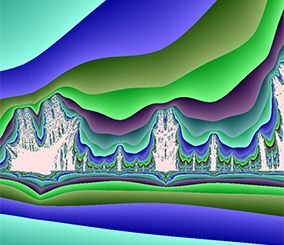Burning ShipBurning ShipBurning Ship

### Burning Ship Escape Time Fractals

The BurningShip command generates Array images which provide a visualization of the BurningShip set. The entries of the image are shaded according to the behavior of complex input points under a particular iterative rational map. Taking an initial point z=0+0*I then for each complex input point c a sequence of points z[i] are computed until abs(z[i]) exceeds the cutoff value (ie. escapes).

How to create a Burning Ship fractal in Maple

Learn about Escape-Time Fractals in MapleJuliaJulia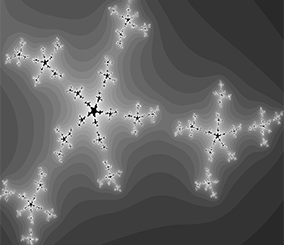JuliaJuliaJulia

### Julia Escape Time Fractals

The Julia command generates Array images which provide a visualization of a Julia set. The entries of the image are shaded according to the behavior of complex input points under a particular iterative rational map characterized by zc a given complex parameter value. For each complex input point z a sequence of points z[i] are computed until abs(z[i]) exceeds the cutoff value (ie. escapes).

How to create a Julia fractal in Maple

Learn about Escape-Time Fractals in MapleLyapunovLyapunovLyapunovLyapunovLyapunov

### Lyapunov Escape Time Fractals

The Lyapunov command generates Array images which provide a visualization of a bifurcational fractal that maps regions of chaos and stability given a binary-valued sequence. The particular binary pattern determines the value of r in the iterative formula.

How to create a Lyapunov fractal in Maple

Learn about Escape-Time Fractals in MapleMandelbrotMandelbrot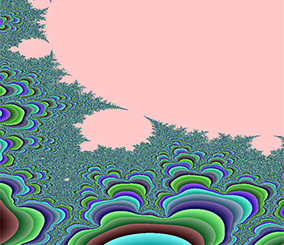MandelbrotMandelbrot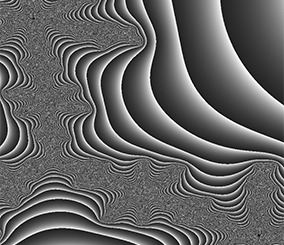MandelbrotMandelbrot

### Mandelbrot Escape Time Fractals

The Mandelbrot command generates Array images which provide a visualization of the Mandelbrot set. The entries of the image are shaded according to the behavior of complex input points under a particular iterative rational map. Taking an initial point z=0+0*I then for each complex input point c a sequence of points z[i] are computed until abs(z[i]) exceeds the cutoff value (ie. escapes).

How to create a Mandelbrot fractal in Maple

Learn about Escape-Time Fractals in MapleNewtonNewtonNewtonNewtonNewton

### Newton Escape Time Fractals

The Newton command generates Array images which provide a visualization of the Newton fractal. The entries of the image are shaded according to the behavior of complex input points under the iterative Newton rootfinding process. For each complex input point z a sequence of points z[i] are computed until either a final point meets a convergence criterion (escapes) or the iteration limit is exceeded.

How to create a Newton fractal in Maple

Learn about Escape-Time Fractals in Maple

## Iterative MapsLorenz AttractorLorenz AttractorLorenz Attractor

### Lorenz Attractor Iterative Maps

The Lorenz system is a system of ordinary differential equations that was originally derived by Edward Lorenz as a simplified model of atmospheric convection.

Interestingly, the evolution of the system for certain values of the parameters and initial conditions exhibits chaotic behavior. In particular the Lorenz attractor is the solution obtained for the Lorenz system with Ρ = 28, σ = 10, and β = 8/3.

Explore the Lorenz System in the MapleCloud

How to create a Lorenz Attractor in Maple

Hopalong Attractor Application

Similar to the fractals, the Lorenz Attactor can be generated by an iterated map process.AttractorAttractorAttractor

### Attractor Iterative Maps

The Attractor command generates an image of points of an iterative function (the first variable in vars against the second variable in vars) being applied iterations times.

How to create an Attractor fractal in Maple

Learn about Iterative Maps in Maple

Similar to the fractals, the Attactor can be generated by an iterated map process.BifurcationBifurcationBifurcation

### Bifurcation Iterative Maps

The Bifurcation module generates an Array image for an iterative function (rvariable against the first variable in vars) being applied iter times, to a domain of equally spaced rvariable values.

How to create an Bifurcation fractal in Maple

Learn about Iterative Maps in Maple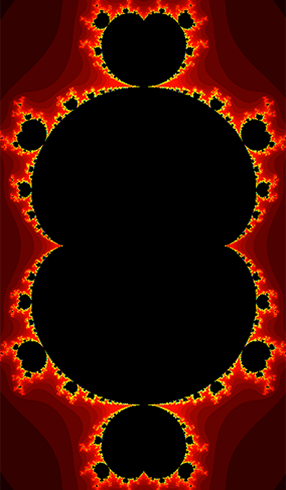Escape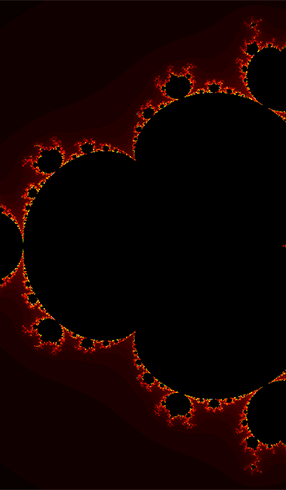Escape

### Escape Iterative Maps

The Escape module is a general purpose mechanism that can also recreate each of the MandelBrot, Julia, Burning Ship, and Newton escape-fractal images in addition to many more variants. More specifically, the Escape module computes the escape values of an iterative function (xvariable against yvariable) being applied iterations times at equally spaced (xvariable,yvariable) values, and constructs an image of the results.